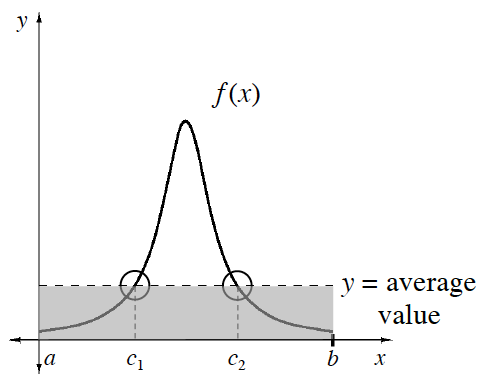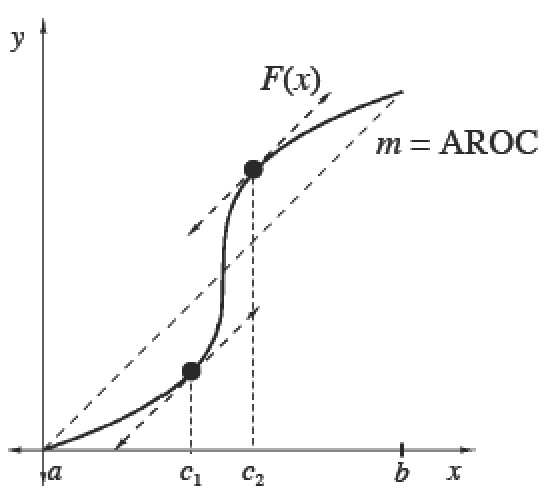### Home > APCALC > Chapter 8 > Lesson 8.1.3 > Problem8-35

8-35.

Is there a $c$ in the interval $[0, 1]$ for $f (x) = x^2 - x$ where $f ^\prime(c) = 0$? Justify your answer.

If $f(x)$ is continuous AND differentiable on the given domain, then the Mean Value Theorem will apply.

The Mean Value Theorem

 The Mean Value Theorem for IntegralsIf $f$  is continuous on $[a, b]$, then there exists at least one point $x = c$ in $\left(a, b\right)$ such that                     $\frac { 1 } { b - a } \int _ { a } ^ { b } f ( x ) d x= f(c)$.The Mean Value Theorem for DerivativesIf $F$ is continuous on $[a, b]$ and differentiable on $\left(a, b\right)$, then there exists at least one point $x = c$ in $\left(a, b\right)$ such that $F ^ { \prime } ( c ) = \frac { F ( b ) - F ( a ) } { b - a } = f ( c )$.